Intelligent Traffic Evaluation and Management System

An intelligent traffic evaluation, management and monitoring system with cloud data analysis.

IntermediateFull instructions provided20 hours184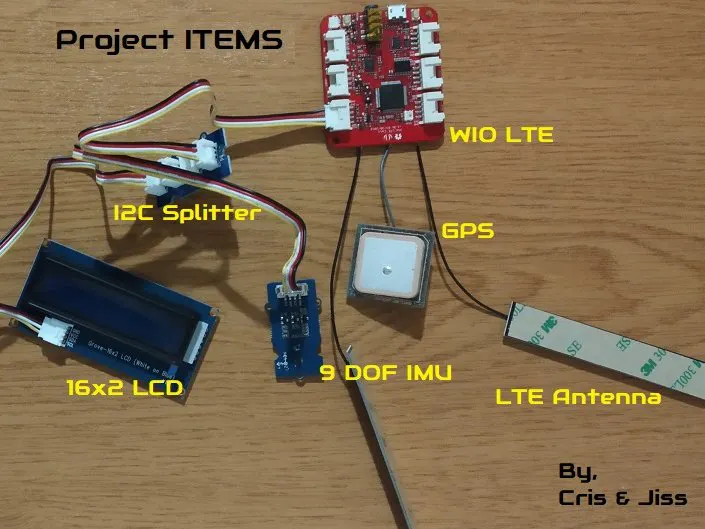Things used in this project

Hardware components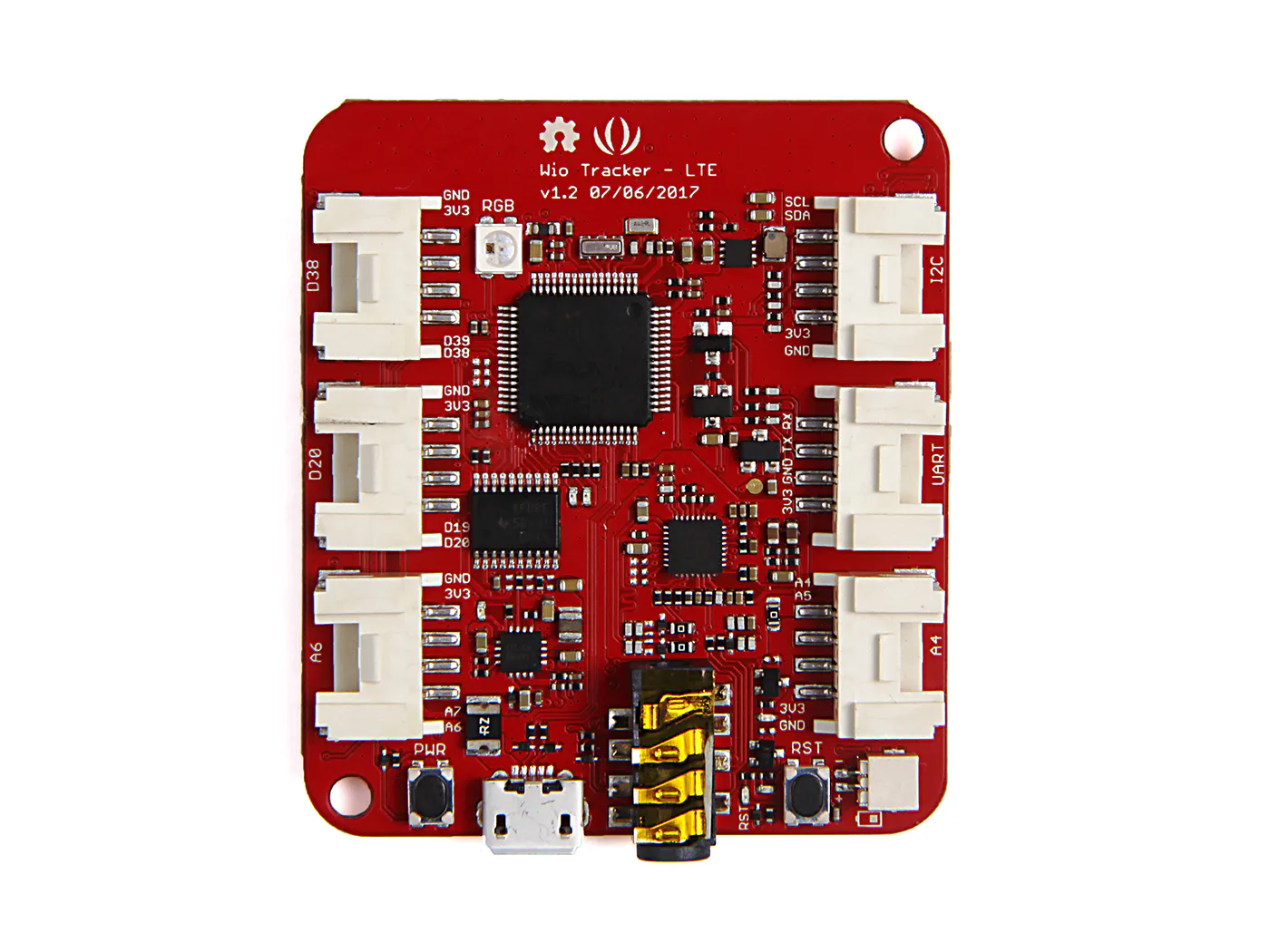Seeed Wio LTE US Version - 4G, Cat.1, GNSS, JavaScript(Espruino) Compatible
×1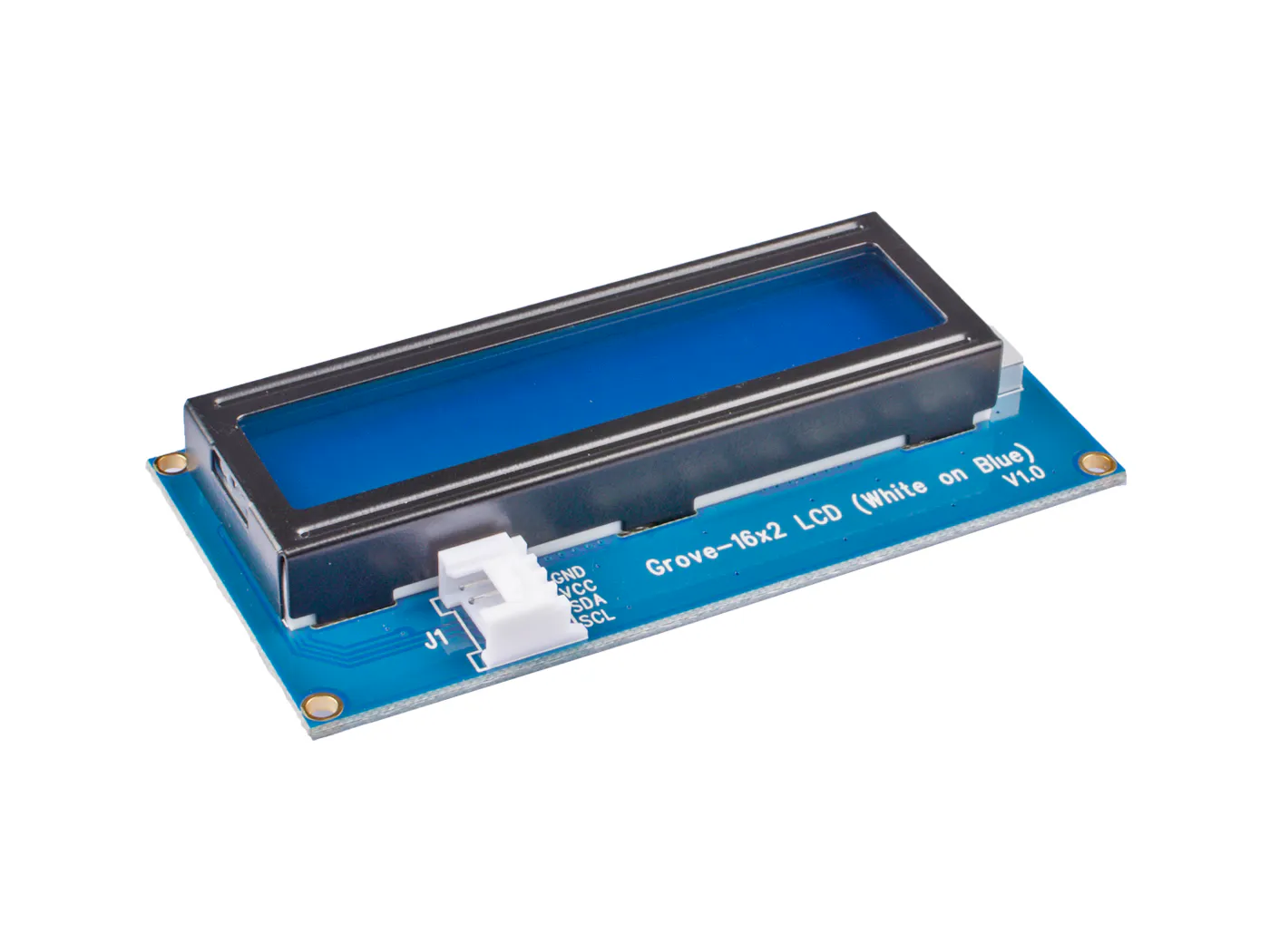Seeed Grove - 16 x 2 LCD (White on Blue)
×1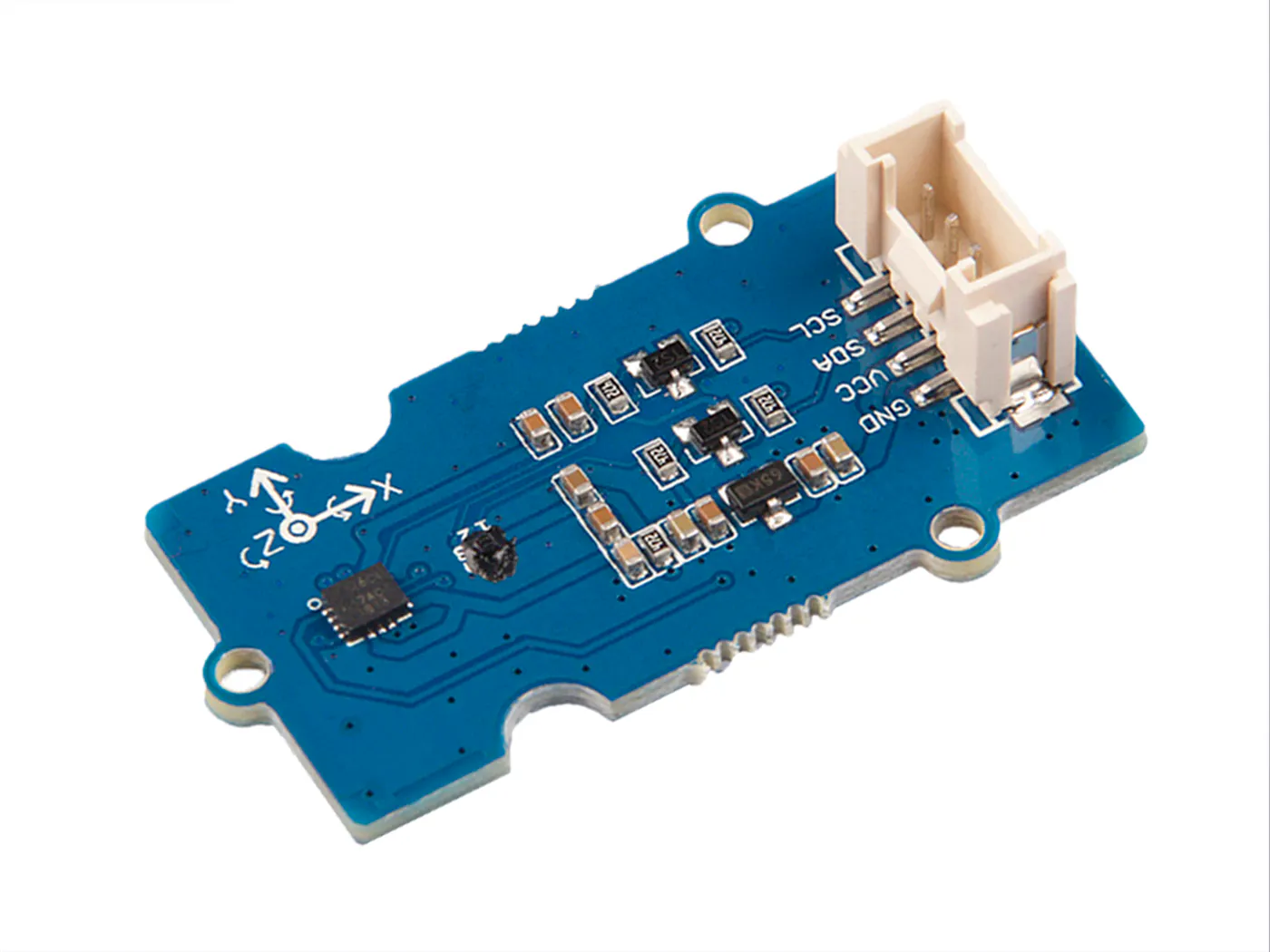Seeed Grove - IMU 9DOF (ICM20600+AK09918)
×1
×1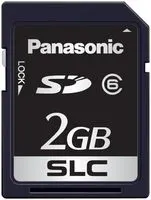Flash Memory Card, SD Card
×1

Software apps and online servicesArduino IDE

Code

Project ITEMS

C/C++
Arduino sketch (given as C/C++ version for readability)
//-------------------------------------------------------------------------------//
//-- PROJECT ITEMS                                                               //
//                                                                               //
//-- PROGRAM GOALS                                                               //
//-- An intelligent Traffic Evaluation, Management and Monitoring system         //
//-- with Cloud data analysis                                                    //
//                                                                               //
//-- Programmers: Cris Thomas, Jiss Joseph Thomas                                //
//-- References: WIO LTE                                                         //
//-- Contact: cris.thomas1@gmail.com, jissdeodates@gmail.com                     //
//-------------------------------------------------------------------------------//

#include "AK09918.h"
#include "ICM20600.h"
#include "gnss.h"
#include "rgb_lcd.h"
#include <Wire.h>
#include <Time.h>
#include <SD.h>

AK09918_err_type_t err;
int32_t x, y, z;
AK09918 ak09918;
ICM20600 icm20600(true);
int16_t acc_x, acc_y, acc_z;
int32_t offset_x, offset_y, offset_z;
double roll, pitch;
double declination_genova = +60.3;
rgb_lcd lcd;
const int chipSelect = 43;
File dataFile;

double vel, vel_x, vel_y, vel_z;
double lat, lon;
int start_t, now_t;
int sd_write = 0;

const int vehicle_id = 1;

void setup()
{
Wire.begin();
start_t = millis();

err = ak09918.initialize();
icm20600.initialize();
ak09918.switchMode(AK09918_POWER_DOWN);
ak09918.switchMode(AK09918_CONTINUOUS_100HZ);
while (err != AK09918_ERR_OK)
{
delay(100);
}
delay(2000);
calibrate(10000, &offset_x, &offset_y, &offset_z);

gnss.Power_On();
while(false == gnss.Check_If_Power_On()){
delay(1000);
}
if(!(gnss.open_GNSS())){
return;
}
delay(2000);

lcd.begin(16, 2);
delay(1000);

pinMode(SS, OUTPUT);
if (SD.begin(chipSelect))
{
sd_write = 1;
dataFile = SD.open("ITEMS_log.txt", FILE_WRITE);
dataFile.println("Project ITEMS");
dataFile.println("by Cris and Jiss");
dataFile.println("");
}

lcd.setCursor(0, 0);
lcd.print("Project ITEMS");
lcd.setCursor(0, 1);
lcd.print("By Cris and Jiss");

delay(1000);

lcd.setCursor(0, 0);
lcd.print("Vehicle ID -->");
lcd.setCursor(0, 1);
lcd.print(vehicle_id);
if(sd_write)
{
dataFile.println("Vehicle ID: " + vehicle_id);
}
}

void loop()
{
// get acceleration
acc_x = icm20600.getAccelerationX();
acc_y = icm20600.getAccelerationY();
acc_z = icm20600.getAccelerationZ();

ak09918.getData(&x, &y, &z);
x = x - offset_x;
y = y - offset_y;
z = z - offset_z;

// roll/pitch in radian
roll = atan2((float)acc_y, (float)acc_z);
pitch = atan2(-(float)acc_x, sqrt((float)acc_y*acc_y+(float)acc_z*acc_z));
roll = roll*57.3;
pitch = pitch*57.3;
double Xheading = x * cos(pitch) + y * sin(roll) * sin(pitch) + z * cos(roll) * sin(pitch);
double Yheading = y * cos(roll) - z * sin(pitch);

delay(500);

// Finding velocity
now_t = millis();
vel_x = vel_x + (acc_x*(now_t - start_t));
vel_y = vel_y + (acc_x*(now_t - start_t));
vel_z = vel_z + (acc_x*(now_t - start_t));
vel = sqrt((vel_x^2) + (vel_y^2));

lcd.setCursor(0, 0);
lcd.print("Velocity -->");
lcd.setCursor(0, 1);
lcd.print(vel + " m/s");
if(sd_write)
{
dataFile.println("Velocity: " + vel);
}

if(gnss.getCoordinate())
{
lon = gnss.longitude;
lat = gnss.latitude;

lcd.setCursor(0, 0);
lcd.print("GNSS : Lat -->");
lcd.setCursor(0, 1);
lcd.print(lat);
if(sd_write)
{
dataFile.println("Lat: " + lat);
}

lcd.setCursor(0, 0);
lcd.print("GNSS : Long -->");
lcd.setCursor(0, 1);
lcd.print(lon);
if(sd_write)
{
dataFile.println("Long: " + lon);
}
}

}

void calibrate(uint32_t timeout, int32_t *offsetx, int32_t *offsety, int32_t*offsetz)
{
int32_t value_x_min = 0;
int32_t value_x_max = 0;
int32_t value_y_min = 0;
int32_t value_y_max = 0;
int32_t value_z_min = 0;
int32_t value_z_max = 0;
uint32_t timeStart = 0;

ak09918.getData(&x, &y, &z);

value_x_min = x;
value_x_max = x;
value_y_min = y;
value_y_max = y;
value_z_min = z;
value_z_max = z;
delay(100);

timeStart = millis();

while((millis() - timeStart) < timeout)
{
ak09918.getData(&x, &y, &z);

if(value_x_min > x)
{
value_x_min = x;
}
else if(value_x_max < x)
{
value_x_max = x;
}

if(value_y_min > y)
{
value_y_min = y;
}
else if(value_y_max < y)
{
value_y_max = y;
}

if(value_z_min > z)
{
value_z_min = z;
}
else if(value_z_max < z)
{
value_z_max = z;
}

Serial.print(".");
delay(100);

}

*offsetx = value_x_min + (value_x_max - value_x_min)/2;
*offsety = value_y_min + (value_y_max - value_y_min)/2;
*offsetz = value_z_min + (value_z_max - value_z_min)/2;
}

Credits

Cris Thomas

7 projects • 39 followers
Electronics and Aerospace engineer with a dedicated history in Research and Development

Jiss Joseph Thomas

5 projects • 29 followers
Co-Researcher Trainee (Artificial Intelligence) at Creopedia Business Intelligence Pvt.Ltd, Kochi,